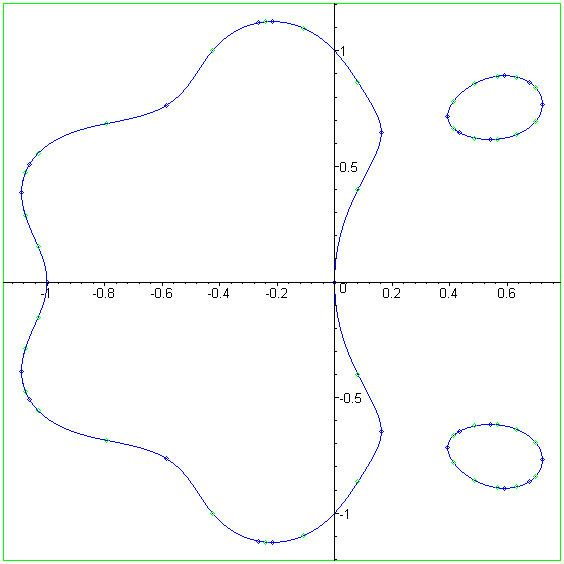# Polynomial lemniscate

Updated on
Covid-19In mathematics, a polynomial lemniscate or polynomial level curve is a plane algebraic curve of degree 2n, constructed from a polynomial p with complex coefficients of degree n.

## Contents

For any such polynomial p and positive real number c, we may define a set of complex numbers by | p ( z ) | = c . This set of numbers may be equated to points in the real Cartesian plane, leading to an algebraic curve ƒ(xy) = c2 of degree 2n, which results from expanding out p ( z ) p ¯ ( z ¯ ) in terms of z = x + iy.

When p is a polynomial of degree 1 then the resulting curve is simply a circle whose center is the zero of p. When p is a polynomial of degree 2 then the curve is a Cassini oval.

## Erdős lemniscate

A conjecture of Erdős which has attracted considerable interest concerns the maximum length of a polynomial lemniscate ƒ(xy) = 1 of degree 2n when p is monic, which Erdős conjectured was attained when p(z) = zn − 1. This is still not proved but Fryntov and Nazarov proved that p gives a local maximum. In the case when n = 2, the Erdős lemniscate is the Lemniscate of Bernoulli

( x 2 + y 2 ) 2 = 2 ( x 2 y 2 )

and it has been proven that this is indeed the maximal length in degree four. The Erdős lemniscate has three ordinary n-fold points, one of which is at the origin, and a genus of (n − 1)(n − 2)/2. By inverting the Erdős lemniscate in the unit circle, one obtains a nonsingular curve of degree n.

## Generic polynomial lemniscate

In general, a polynomial lemniscate will not touch at the origin, and will have only two ordinary n-fold singularities, and hence a genus of (n − 1)2. As a real curve, it can have a number of disconnected components. Hence, it will not look like a lemniscate, making the name something of a misnomer.

An interesting example of such polynomial lemniscates are the Mandelbrot curves. If we set p0 = z, and pn = pn−12 + z, then the corresponding polynomial lemniscates Mn defined by |pn(z)| = 1 converge to the boundary of the Mandelbrot set. The Mandelbrot curves are of degree 2n+1.

Topics